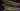# Coding Interview - Useful Terms Cheatsheet

May 16, 2019## Big-O notation

In simpler terms, its kind of a unit to measure how efficient an algorithm is, with respect to the size of input, often refered to as ‘n’. There are two kind of units in algorithms:

1. Time Complexity It defines how much time an algorithm is taking w.r.t. ‘n’
2. Space Complexity It defines much space an algorithm is taking w.r.t. ‘n’

In interviews, mostly people are interested in Time complexities, but you should be familiar with space complexitity as well.

For example, Bubble Sort’s time complexity is O(n^2) What it means, if the number of input is: 10. This algorithm requires an average of 10^2=100 operations or CPU cycles to finish working.

Where as, Merge Sort takes O(n log n). Which is clearly lesser than O(n^2). So, lesser the complixity, more efficient the algorithm, i.e. more quickly it can be finished.

Note: here log n means log (base 2) of n

## Divide and Conquer Algorithms

These algorithms are very useful and recursive in nature. These algorithms break the given input set or given problem in smaller sets or smaller subproblems. Then, solve the subproblems recursively, and finally combine these solutions to have a final solution for original problem.

To be more precise:

### Divide

Divide the problem into smaller sub-problems. Its like big problem is now converted into smaller problems. And it is relatively easy to solve a smaller problem rather than bigger problem.

### Conquer

Conquer the subproblems by solving them recursively.

### Combine

Combine the solution of smaller sub-problems which becomes solution to original bigger problem.

Merge sort, Quick sort are best example of this approach. Once you learn the basic concept behind this, rest of the problems become very easy for you.

## Stable Sort

In sorting, the numbers with the same value appear in the output array in the same order as they do in the input array. For same value numbers/objects, the order among them will remain same.